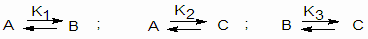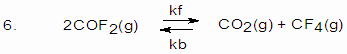CHEMISTRY

 Time: 1 Hour Max.Marks : 60

Instructions:

(1) Answer must be written either in English or the medium of instruction of the candidate in high school.
(2) There will be no negative marking
(3) Use of calculators or graph papers is not permitted

 I. Explain the following 5x3=15M

1. The value of K (equilibrium constant, rate constant, solubility constant) changes with temperature. But radioactive decay constant is independent of temperature.
2. On expose to air, caustic soda becomes liquid and after some time it changes to white powder.
3. Aqueous solution of AlCl3 behaves acidic towards litmus while that of NaCl not.
4. Both coke and flux are used in smelting process of metallurgy.
5. Bottle containing NaOH solution should not be closed by glass lid.

 II. Differentiate the following: 5x3=15M

1. An equivalent in acid-base reaction and an equivalent in oxidation–reduction reaction
2. Acid strength and concentration
3. Mixed salt and Double salt
4. Complex salt and Simple salt
5. Contrast the action of heat on the following (with equations)
(a) Na2CO3 and CaCO3
(b) MgCl2.6H2O and CaCl2.6H2O
(c) Ca(NO3)2 and NaNO3

1. A fuel gas contains carbon and hydrogen only. Burning a small sample of it in oxygen gives 3.38 gm carbon dioxide, 0.69 gm of water and no other products. A volume of 10 lit (measured at STP) of this gas is found to weigh 11.6 gm.

(a) Calculate
(i) empirical formula
(ii) molecular mass of the gas
(iii) molecular formula
(b) Write one single step preparation of the gas
(c) Does it react with bromine? If so what colour change do you expect?
(d) What do you expect the calorific value of the gas with reference to marsh gas?

2. At a given temperature, the degree of ionization (fraction dissociated) of water is found to be 1.8x10-9. Calculate the ionization constant and the ionic product of water at this temperature.

3. Suppose the gas-phase isomerisation reactionsreach equilibrium at a fixed temperature. Express the equilibrium mole fractions of A,B and C in terms of equilibrium constants, K1, K2 and K3.

4. A sample of P2O5 contains some impurity. 0.405 gm sample is reacted with water and the resulting solution requires 42.5 ml of 0.25 M NaOH solution. The salt resulted is monobasic acid salt. Calculate the percent impurity.

5. What will be the resultant pH when 200 ml of an aqueous solution of HCl (pH=2) is mixed with 300 ml of an aqueous solution of NaOH (pH=12).K=2 for the reaction at 10000C.

If a 5L mixture contains 0.105 mole COF2, 0.22 mol CO2 and 0.055 mol CF4 at 10000C.

(a) Will the mixture be at equilibrium?
(b) If the gases are not at equilibrium, in which direction will a net reaction occur?
(c) What is the amount of each gas present when equilibrium is established.
(d) What are the rate equations for the forward and backward reactions?
(e) Compare the rate constants at (i) initial stage and (ii) equilibrium stage

7. For the reaction

(i) A B ; Kc = 2
(ii) B C ; Kc = 4
(iii) C D ; Kc = 6

For the reaction A D what is the Kc?

8. Four elements coded A,B,C and D form a series of substances e.g. AB, B2, CB3, DB2 and DB3. If the atomic number of these elements are not necessarily in the order are 13, 19, 26 and 35. Write down extra nuclear electronic structures of these elements. From this information and the formula of the compounds, allocate A,B,C and D with appropriate atomic numbers. Mention the nature of bonding in each of them.

9. Calculate the molecular weight of hydrogen fluoride if density of this gas is 3.17 g/L at 300K and 1.0 atm pressure. What information can you deduce from the result?

10. Anhydrous copper sulphate turns to blue from colourless on expose to atomosphere and again make it colourless by some substance (X). When copper sulphate is added to water a blue colour solution is resulted with some turbidity. The turbidity disappears by the addition of a drop of HCl solution. On placing Iron rod in it, a greenish solution is resulted. A chocolate like precipitate is resulted when a drop of potassium ferrocyanide solution is added to the blue colour solution of copper sulphate. Black precipitate is resulted by passing H2S gas through copper sulphate solution but the precipitate is soluble in conc.HNO3.
(a) What is X ?
(b) Write the chemical reactions to explain the observations.